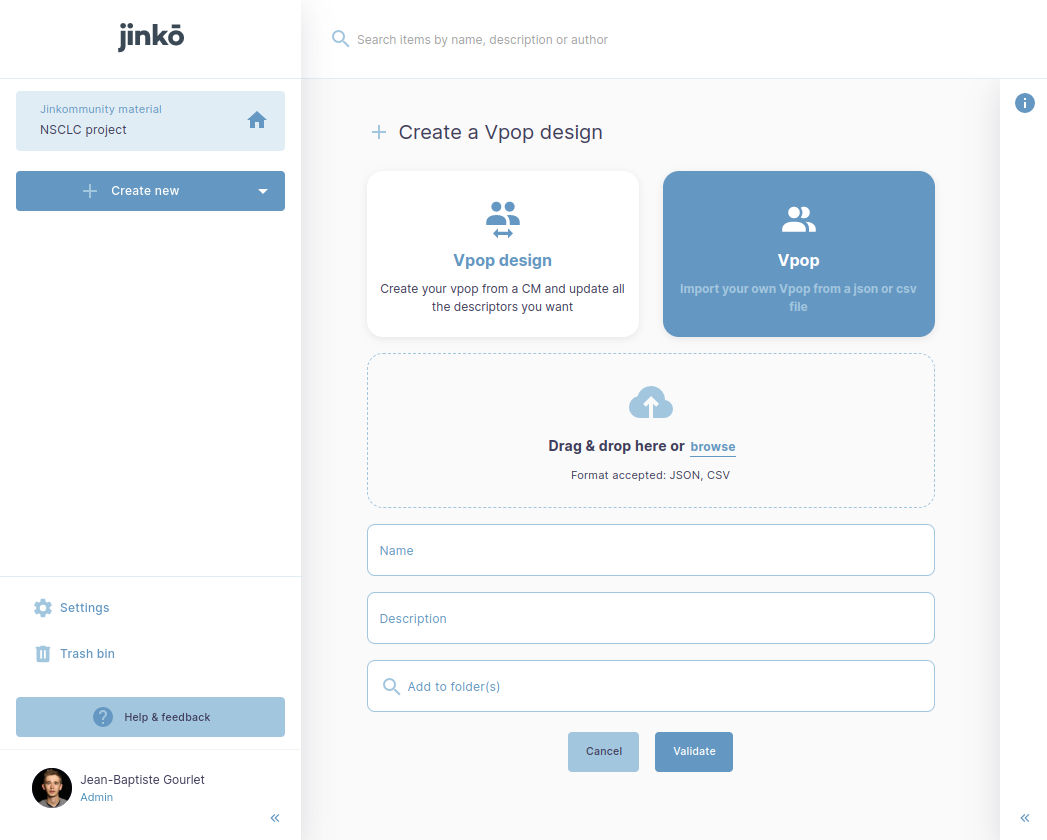1

# How-to upload a virtual population?

• 8 mths ago

You can upload your population in jinkō with two different formats (JSON and CSV). This can be done using the create VPOP feature## Requirements

In both cases (JSON  and CSV), you need the following information per patient:

• A patientIndex: Text - a unique value identifying the patient

• A set of patientAttributes where each attribute has:

• An identifier

• A value

• An optional unit

This translates as follows for each format:

### CSV format

The separator is the standard comma: “,”

The header follows the pattern patientIndex,id1(unit1), id2, ..., idn(unitn) . The unit can be specified in brackets (unit) after the id. In this example id2 does not have a unit specified

Body follows the following pattern: patient1, val11, val12, ..., val1n

• patientIndex column: Text, should be unique for each row

• Patient descriptors columns: Float is mandatory

Example (presented in tabular format for readability):

 patientIndex age(year) sizeHelper isMale patient1 60.7 0.294 1 patient2 55.2 0.407 0 patient3 70.3 0.822 0

### JSON format

The virtual population object is a list of patients

Each Patient has:

• A "patientIndex": Text

• A "patientAttributes": Object

• "id": Text

• "val": Float

• "unit": Text (optional)

Example:

``````{
"patients": [{
"patientIndex": "patient1",
"patientAttributes": [{
"id": "age",
"val": 60.7,
"unit": "year"
},
{
"id": "sizeHelper",
"val": 0.294,
"unit": "dimensionless"
},
{
"id": "isMale",
"val": 1,
"unit": "dimensionless"
}
],
"patientIndex": "patient1"
},
{
"patientIndex": "patient2",
"patientAttributes": [{
"id": "age",
"val": 55.2,
"unit": "year"
},
{
"id": "sizeHelper",
"val": 0.407,
"unit": "dimensionless"
},
{
"id": "isMale",
"val": 0,
"unit": "dimensionless"
}
]

},
{
"patientIndex": "patient3",
"patientAttributes": [{
"id": "age",
"val": 70.3,
"unit": "year"
},
{
"id": "sizeHelper",
"val": 0.822,
"unit": "dimensionless"
},
{
"id": "isMale",
"val": 0,
"unit": "dimensionless"
}
]

}
]
}``````

## A note on Units

The base units used in the platform are:

•  [length]              :  "meter   = m  = metre"

•  [time]                 : "second  = s    = sec"

•  [current]            : "ampere = A   = amp"

•  [luminosity]      : "candela = cd = candle"

•  [mass]               : "gram     = g"

•  [substance]      : "mole      = mol"

•  [temperature ] : "degK      = K  = kelvin"

•  []                        : "dimensionless”

You can use prefix in the units combination, below is a non-exhaustive of accepted prefix:

• "nano-  = 1e-9  = n-"

• "micro- = 1e-6  = u-"

• "milli-    = 1e-3  = m-"

• "centi-   = 1e-2  = c-"

• "deci-    = 1e-1  = d-"

• "deca-   = 1e+1 = da-"

• "hecto-  = 1e2   = h-"

• "kilo-      = 1e3   = k-"

• "mega-  = 1e6   = M-"

• "giga-    = 1e9   = G-"

You can combine and exponent units as follow:  `kilogram * meter / second ** 2`

• Oldest first
• PopularLike1 Follow
• 1 Likes
• 8 mths agoLast active
• 33Views
• 1 Following

Don't have a jinkō account yet?

-> Request a demo today :)